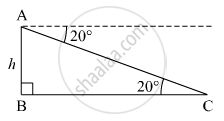Advertisement Remove all ads

# While Landing at an Airport, a Pilot Made an Angle of Depression of 20°. Average Speed of the Plane Was 200 Km/Hr. the Plane Reached the Ground After 54 Seconds. Find the Height at - Geometry

Advertisement Remove all ads
Advertisement Remove all ads
Advertisement Remove all ads

While landing at an airport, a pilot made an angle of depression of 20°. Average speed of the plane was 200 km/hr. The plane reached the ground after 54 seconds. Find the height at which the plane was when it started landing. (sin 20° = 0.342)

Advertisement Remove all ads

#### Solution

Let the plane was at a height of h m when it started landing.
Average speed of the plane = 200 km/h = $200 \times \frac{5}{18} = \frac{500}{9}$ m/s
Time taken by plane to reach the ground = 54 s
∴ Distance covered by the plane to reach the ground = Average speed of the plane × Time taken by plane to reach the ground
= $\frac{500}{9} \times 54$
= 3000 mHere, AC = 3000 m and ∠ACB = 20º
In right ∆ABC,
$\sin20^\circ = \frac{AB}{AC}$
$\Rightarrow 0 . 342 = \frac{h}{3000}$
$\Rightarrow h = 0 . 342 \times 3000 = 1026 m$

Thus, the plane was at the height of 1026 m when it started landing.

Concept: Heights and Distances
Is there an error in this question or solution?

#### APPEARS IN

Balbharati Mathematics 2 Geometry 10th Standard SSC Maharashtra State Board
Chapter 6 Trigonometry
Problem Set 6 | Q 10 | Page 139

#### Video TutorialsVIEW ALL 

Advertisement Remove all ads
Share
Notifications

View all notifications

Forgot password?